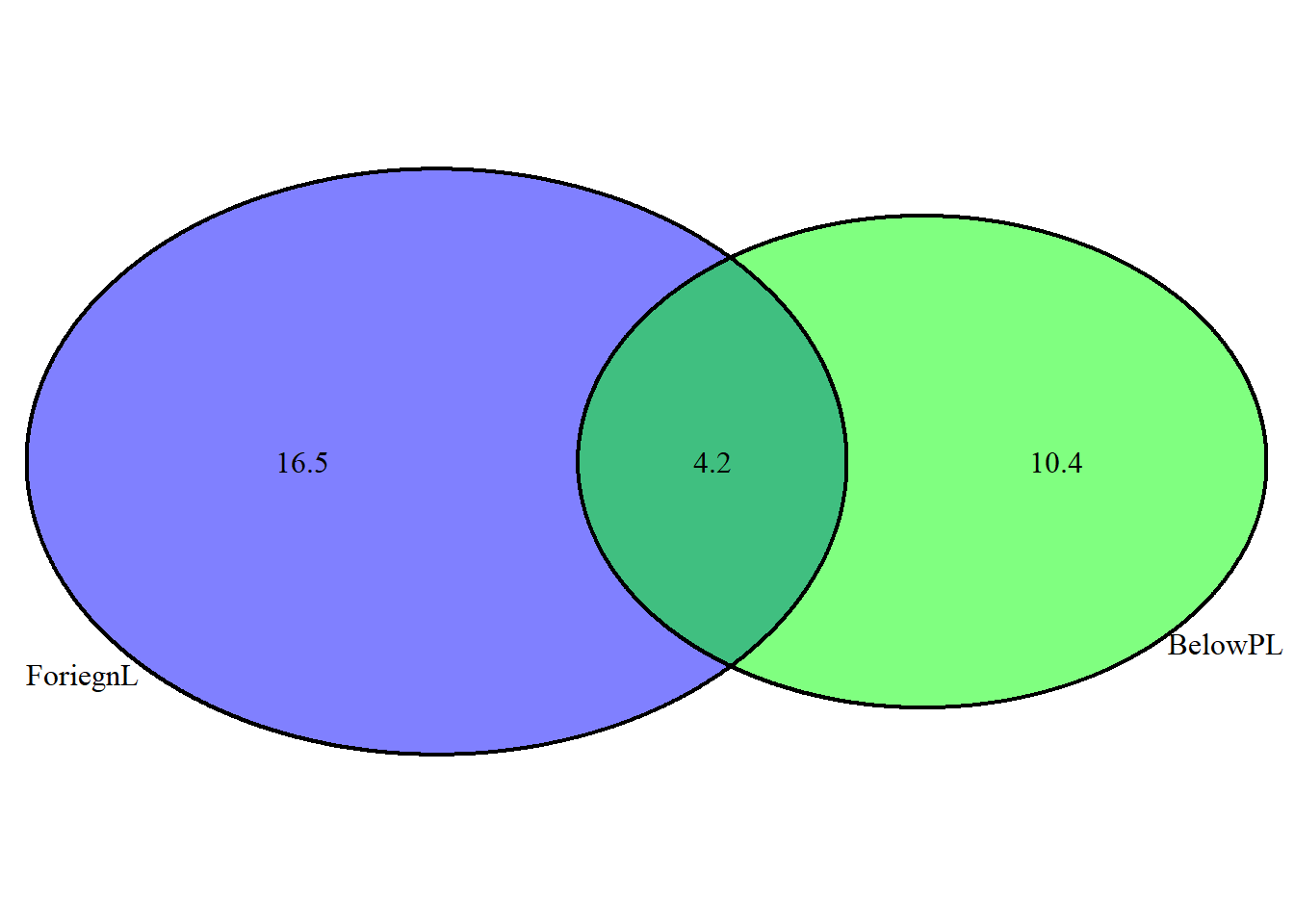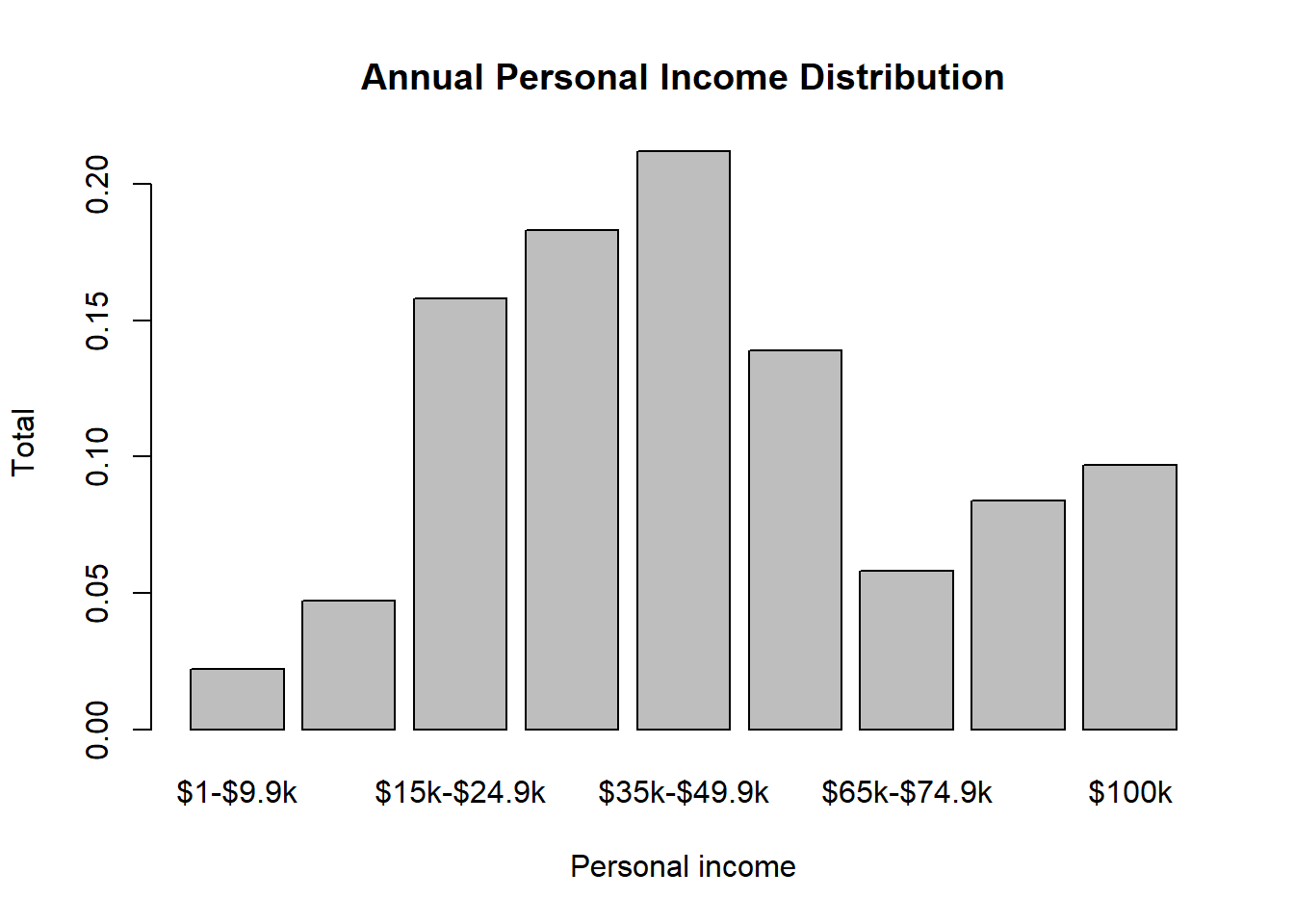Dice rolls. (3.6, p. 92) If you roll a pair of fair dice, what is the probability of

# two dice roll in a matrix
dice1 <- c(1,2,3,4,5,6)
dice2 <- c(1,2,3,4,5,6)
dicesum<-matrix(c(dice1+dice2,dice1+dice2,dice1+dice2,dice1+dice2,dice1+dice2,dice1+dice2),nrow = 6, byrow=TRUE)
dicesum
##      [,1] [,2] [,3] [,4] [,5] [,6]
## [1,]    2    3    4    5    6    7
## [2,]    3    4    5    6    7    8
## [3,]    4    5    6    7    8    9
## [4,]    5    6    7    8    9   10
## [5,]    6    7    8    9   10   11
## [6,]    7    8    9   10   11   12
1. getting a sum of 1?

Can not have sum of 1 by rolling two fair dice. Minimum sum you can get is 2 according to the sum matrix.

1. getting a sum of 5?

According to the sum matrix there are 4 possible chances of getting sum of 5. (4,1), (3,2), (2,3), (1,4):

#Possibility of getting sum of 5
sumof5_tot_chances <- 4
tot_Possible_outcomes <- 36
Pof5 <- sumof5_tot_chances/tot_Possible_outcomes
Pof5
##  0.1111111
1. getting a sum of 12?

According to the sum matrix there are only one possible chance of getting sum of 12. (6,6):

#Possibility of getting sum of 12
sumof12_tot_chances <- 1
Pof12 <- sumof12_tot_chances/tot_Possible_outcomes
Pof12
##  0.02777778

Poverty and language. (3.8, p. 93) The American Community Survey is an ongoing survey that provides data every year to give communities the current information they need to plan investments and services. The 2010 American Community Survey estimates that 14.6% of Americans live below the poverty line, 20.7% speak a language other than English (foreign language) at home, and 4.2% fall into both categories.

1. Are living below the poverty line and speaking a foreign language at home disjoint?

As 4.2% fall into both categories, Both events can happen at the same time. it is not disjoint or mutually exclusive.

1. Draw a Venn diagram summarizing the variables and their associated probabilities.
library(VennDiagram)
## Loading required package: grid
## Loading required package: futile.logger
PL <- 14.6/100
FL <- 20.7/100
PLandFL <- 4.2/100

grid.newpage()
draw.pairwise.venn(area1 = 14.6, area2 = 20.7, cross.area = 4.2, category = c("BelowPL", "ForiegnL"), fill = c("green","blue"))## (polygon[GRID.polygon.1], polygon[GRID.polygon.2], polygon[GRID.polygon.3], polygon[GRID.polygon.4], text[GRID.text.5], text[GRID.text.6], text[GRID.text.7], text[GRID.text.8], text[GRID.text.9])
1. What percent of Americans live below the poverty line and only speak English at home?
(PL - PLandFL)*100
##  10.4

Based on the venn diagram 10.4% Americans live below the poverty line and only speak English at home

1. What percent of Americans live below the poverty line or speak a foreign language at home?
((PL + FL)- PLandFL) *100
##  31.1

31.1% of Americans live below the poverty line or speak a foreign language at home

1. What percent of Americans live above the poverty line and only speak English at home?
100 - (((PL + FL)- PLandFL) *100)
##  68.9

68.9% Americans live above the poverty line and only speak English at home

1. Is the event that someone lives below the poverty line independent of the event that the person speaks a foreign language at home?

It is not independent. Event speaks a foreign language at home can falls within the event below the poverty line.

Assortative mating. (3.18, p. 111) Assortative mating is a nonrandom mating pattern where individuals with similar genotypes and/or phenotypes mate with one another more frequently than what would be expected under a random mating pattern. Researchers studying this topic collected data on eye colors of 204 Scandinavian men and their female partners. The table below summarizes the results. For simplicity, we only include heterosexual relationships in this exercise.

1. What is the probability that a randomly chosen male respondent or his partner has blue eyes?

FBlue = 108, MBlue = 114, FMBlue = 78

P_FBlue <- 108/204
P_MBlue <- 114/204
P_FandMBlue <- 78/204
P_FBlue
##  0.5294118
P_MBlue
##  0.5588235
P_FandMBlue
##  0.3823529
#probability that a randomly chosen male respondent or his partner has blue eyes
P_ForMBlue <- (P_FBlue + P_MBlue) -  P_FandMBlue
P_ForMBlue
##  0.7058824
1. What is the probability that a randomly chosen male respondent with blue eyes has a partner with blue eyes?
P_FandMBlue/P_MBlue
##  0.6842105
1. Whatistheprobabilitythatarandomlychosenmalerespondentwithbrowneyeshasapartner with blue eyes? What about the probability of a randomly chosen male respondent with green eyes having a partner with blue eyes?

MBrown = 54, MGreen = 36

#robability that arandomly chosen male respondent with brown eyes has apartner with blue eyes
P_MBrwonFBlue <- 19/54
P_MBrwonFBlue
##  0.3518519
#probability of a randomly chosen male respondent with green eyes having a partner with blue eyes
P_MGreenFBlue <- 11/36
P_MGreenFBlue
##  0.3055556
1. Does it appear that the eye colors of male respondents and their partners are independent? Explain your reasoning.

These are independent as one event does not provide any useful information on other event. Eye color of male do not provide the eye color of their partners.

Books on a bookshelf. (3.26, p. 114) The table below shows the distribution of books on a bookcase based on whether they are nonfiction or fiction and hardcover or paperback.

1. Find the probability of drawing a hardcover book first then a paperback fiction book second when drawing without replacement.
P_HC <- 28/95
P_PBF <- 59/94
# probability of drawing a hardcover book first then a paperback fiction book second when drawing without replacement
(P_HC)*(P_PBF)
##  0.1849944
1. Determine the probability of drawing a fiction book first and then a hardcover book second, when drawing without replacement.
P_FB <- 72/95
P_HC <- 28/94
(P_FB)*(P_HC)
##  0.2257559
1. Calculate the probability of the scenario in part (b), except this time complete the calculations under the scenario where the first book is placed back on the bookcase before randomly drawing the second book.
P_FB <- 72/95
P_HC <- 28/95
(P_FB)*(P_HC)
##  0.2233795
1. The final answers to parts (b) and (c) are very similar. Explain why this is the case.

As population of these two events are very large, sampling with replacement isn’t much differnence from sampling without replacement.

Baggage fees. (3.34, p. 124) An airline charges the following baggage fees: $25 for the first bag and$35 for the second. Suppose 54% of passengers have no checked luggage, 34% have one piece of checked luggage and 12% have two pieces. We suppose a negligible portion of people check more than two bags.

1. Build a probability model, compute the average revenue per passenger, and compute the corresponding standard deviation.
baggage_fee <- c(0, 25, 60)
Total_Passengers <- c(54,34, 12)
Luggage <- data.frame(baggage_fee, Total_Passengers)

Luggage
##   baggage_fee Total_Passengers
## 1           0               54
## 2          25               34
## 3          60               12
# Average Revenue per person
avg_revenue <- (sum(Luggage$baggage_fee*Luggage$Total_Passengers))/sum(Luggage$Total_Passengers) avg_revenue ##  15.7 # Standard Deviation baggage_variance <- baggage_fee - avg_revenue baggage_EVariance <- baggage_variance^2 * (Total_Passengers/100) variance2 <- sum(baggage_EVariance) sd <- variance2^(1/2) sd ##  19.95019 1. About how much revenue should the airline expect for a flight of 120 passengers? With what standard deviation? Note any assumptions you make and if you think they are justified. # Expected Revenue for 120 passengers avg_revenue * 120 ##  1884 Income and gender. (3.38, p. 128) The relative frequency table below displays the distribution of annual total personal income (in 2009 inflation-adjusted dollars) for a representative sample of 96,420,486 Americans. These data come from the American Community Survey for 2005-2009. This sample is comprised of 59% males and 41% females. 1. Describe the distribution of total personal income. income <- c("$1-$9.9k", "$10k-$14.9k","$15k-$24.9k", "$25k-$34.9k", "$35k-$49.9k", "$50k-$64.9k", "$65k-$74.9k", "$75k-$99.9k", "$100k")
Tot_perc <- c(0.022, 0.047, 0.158, 0.183, 0.212, 0.139, 0.058, 0.084, 0.097)
income_distribution <- data.frame(income, Tot_perc)
income_distribution
##        income Tot_perc
## 1    $1-$9.9k    0.022
## 2 $10k-$14.9k    0.047
## 3 $15k-$24.9k    0.158
## 4 $25k-$34.9k    0.183
## 5 $35k-$49.9k    0.212
## 6 $50k-$64.9k    0.139
## 7 $65k-$74.9k    0.058
## 8 $75k-$99.9k    0.084
## 9       $100k 0.097 barplot(income_distribution$Tot_perc, names.arg = income, xlab = "Personal income", ylab = "Total", main = "Annual Personal Income Distribution")This has a bimodal distribution.

1. What is the probability that a randomly chosen US resident makes less than $50,000 per year? #probability that a randomly chosen US resident makes less than$50,000 per year
P_less_than_50000 <- paste(round(sum(0.022+0.047+0.158+0.183+0.212)*100, digits = 2), "%")
P_less_than_50000
##  "62.2 %"
1. What is the probability that a randomly chosen US resident makes less than $50,000 per year and is female? Note any assumptions you make. female_less_50000 <- sum(0.022+0.047+0.158+0.183+0.212)*(41/100) female_less_50000 ##  0.25502 1. The same data source indicates that 71.8% of females make less than$50,000 per year. Use this value to determine whether or not the assumption you made in part (c) is valid.
sum(0.718*(41/100))
##  0.29438

While both anwers are very close but not the same, these two events are independant.